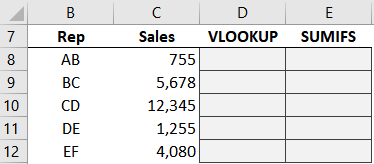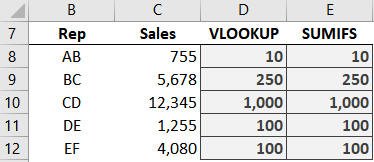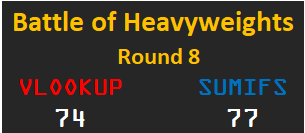# Battle of Heavyweights: Round 8The purpose of this round is to examine the ability of each function to perform a range lookup. A range lookup is where we aren’t looking for an exact matching value, but a value that falls between a range of values. So let’s get to it.

## Round 8

Each sales reps earns a bonus based on their sales volume. So, we grab the sales for each rep:Now, we need to use formulas to retrieve the corresponding bonus amounts from this table (Table1):First, with VLOOKUP.

## VLOOKUP

We write the following formula into D8:

`=VLOOKUP(C8, Table1, 3, TRUE)`

We fill the formula down, and we are looking good:Note: as a reminder, VLOOKUP is looking in the first column only, so, it is only considering the From column when it is trying to find the matching row. Once it does, then it shoots to the right to return the related value. This means that VLOOKUP does not need the To column, and in fact, ignores To completely in this formula.

Now, with SUMIFS.

## SUMIFS

We write the following formula into E8:

`=SUMIFS(Table1[Bonus], Table1[From], "<="&C8, Table1[To], ">="&C8)`

We fill it down:Yes … it worked! But, we need to examine the formula arguments a bit closer.

Let’s break down each argument:

• Table1[Bonus] is the sum range, the Bonus column
• Table1[From] is the first criteria range, the From column
• “<=”&C8 is the first criteria value, and this means that the bonus schedule’s From sales value must be less than or equal to the rep’s sales amount. Note: we enclose the comparison operator <= inside quotes, and then use the concatenation operator & to join it to the criteria value.
• Table1[To] is the second criteria range, the To column
• “>=”&C8 is the second criteria value, and this means that the bonus schedule’s To sales value must be greater than or equal to the rep’s sales amount

So, both functions are able to perform a range lookup … time to analyze the round.

## Analysis

In this round, we wanted to explore the idea of range lookups. So, which one would we use in practice? I think it depends on the workbook and our objective. For example, if we wanted to perform a range lookup and return a text value, we’d use VLOOKUP. If our data had only one lookup column (instead of both the From and To columns), we’d go with VLOOKUP. If we were doing a range lookup and wanted to return the sum of multiple matching rows, we’d go with SUMIFS.

But, in general, I’d have to give the round to VLOOKUP because it is more flexible (can return text, and only needs a single lookup column) and is less complicated to write (no comparison operators and concatenation). So we’ll give 10 points to VLOOKUP. How do we score SUMIFS? Well, SUMIFS was able to do this with the help of the concatenation operator, so we award 9 points.See you in the next post!

Sample file: Round8.xlsx

Get a quick email notice when a new Excel article is available

• This field is for validation purposes and should be left unchanged.1.Tom Tomlinson
1.Jeff Lenning Post author